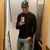# QlikView App Dev

Discussion Board for collaboration related to QlikView App Development.

Announcements
On May 18th at 10AM EDT we will answer your QlikView questions live. REGISTER
cancel
Showing results for
Did you mean:Contributor III

## Set yesterday's date (latest weekday)

I need to create a variable that gives me yesterday's date (in the date format YYYY-MM-DD). When "today" is a Monday (2019-05-20), then the last date prior to that was 2019-05-19, but that is obviously a Sunday, which in most cases is not a workday. Is there a simple statement in the load script or a function that gives it the last workday's date (in this case I would like it to return the date 2019-05-17)?

Thanks

2 Solutions

Accepted SolutionsMVP

Yes the function you need is  FirstWorkDate(). Try like:

FirstWorkDate(Today(),2)Contributor III

i will give a very simple solution:

let vtoday = '2019-05-20'; /// its better if use number - exemple: 43605 represents the same date

let vlastdate = \$(vtoday)-1;

if num(weekday(\$(vlastdate)))=6 then //sunday

let vlastdate = \$(vlastdate)-2;

end if

if num(weekday(\$(vlastdate)))=5 then //saturday

let vlastdate = \$(vlastdate)-1;

end if

trace \$(vlastdate);

exist another ways to calculate the workday...

see help for comand below:

sorry my english,

Andre

4 RepliesMVP

Yes the function you need is  FirstWorkDate(). Try like:

FirstWorkDate(Today(),2)Contributor III

i will give a very simple solution:

let vtoday = '2019-05-20'; /// its better if use number - exemple: 43605 represents the same date

let vlastdate = \$(vtoday)-1;

if num(weekday(\$(vlastdate)))=6 then //sunday

let vlastdate = \$(vlastdate)-2;

end if

if num(weekday(\$(vlastdate)))=5 then //saturday

let vlastdate = \$(vlastdate)-1;

end if

trace \$(vlastdate);

exist another ways to calculate the workday...

see help for comand below:

sorry my english,

AndreContributor III
Author

Thank you Andre.

I think the previous poster solved my problem with a little bit easier function.

If that doesn't work, I will try your solution.

/MathiasContributor III
Author

Thank you for your response. It seems like it is doing the trick. I did some checks by changing the values, and it seemed to change it once there was a weekend the day before. I will see on Monday if it does the trick.

/Mathias